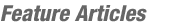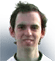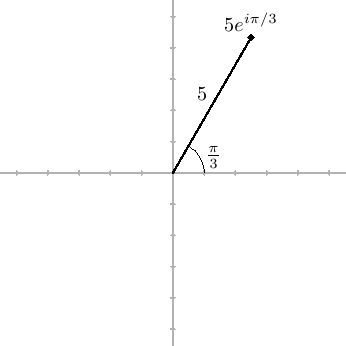JOINComputational geometry with complex numbersBy bmerry
TopCoder Member

Introduction
Computational geometry problems appear frequently in Topcoder challenges and a series of articles has already been written on the subject. However, competitors who have tried to implement these algorithms (particularly in C++) will no doubt have been frustrated by the lack of a built-in point class that provides all the suitable operator overloading, dot and cross products and so on. In fact, there is a class that does most of what one wants, but it is not an obvious choice: the complex number class. In this article I will show how many primitive geometric operations can be quickly translated into the language of complex numbers, and hence into succinct yet readable code.

I am going to assume a familiarity with the basics of computational geometry, as discussed in the article linked above. I will provide a very quick introduction to complex numbers, but the interested reader is advised to read more online or in a mathematics textbook.

Review of complex numbers
Even if you're not familiar with complex numbers, you have probably heard that it has something to do with i, the square root of −1 — an apparently impossible concept. The solution is to treat i as if it were a variable, like x, except that wherever you have i2 you can replace it with −1. Not all the properties of real numbers carry over (in particular, there is no way to order the complex numbers that behaves nicely with respect to the arithmetic operations), but arithmetic properties like commutativity work just the same.

Because powers of i cancel out, all complex numbers can in fact be written in the form a + bi, where a and b are real numbers. Complex numbers can be visualised by plotting them in a plane (called the Argand plane) by using a as the x coordinate (also known as the real part) and b as the y coordinate (also known as the imaginary part), as shown below.Arithmetic operations follow the usual rules, with i2 replaced by −1 as described above. Hence
(a + bi) + (c + di) = (a + c) + (b + d)i
(a + bi)(c + di) = ac + bci + adi + bdi2 = (acbd) + (ad + bc)i
Polar form
An alternative and very important representation for complex numbers is the polar or modulus-argument form. In the Argand plane, this corresponds to a description using the length and direction of the vector connecting 0 to the complex number. For various technical reasons, this is written as
reiθ = r(cos θ + isin θ),
as shown in the figure below. In this form, r is called the modulus (or absolute value) and θ is called the argument, and is always in radians. The modulus of z is written |z|.The polar form leads to Euler's famous equation e + 1 = 0.

Complex conjugate
If z = a + bi, then the conjugate of z is z = a − bi. It has the useful properties that
• z + z = 2a
• zz = 2bi
• zz = a2 + b2 = |z|2
In the polar form, taking a conjugate negates the angle.

Geometric interpretation
Quite conveniently, the complex numbers form a vector space over the real numbers. Put plainly, this means that addition, subtraction, negation and scalar multiplication (i.e., multiplication by a real number) all act on complex numbers in exactly the same way they act on vectors. The action of conjugation is also easy to fathom: it reflect the number in the real axis.

Complex multiplication is less obvious, and does not correspond to any vector operation. However, the obscure exponential in the polar form provides a hint: the modulii are multiplied, but the arguments are added.Putting it all to use
C++ complex number template
C++ has a complex number template, which implements all the operations described above, plus a few more. It takes a template parameter, which is the underlying type for the real and imaginary parts. While it is really intended to be used with floating point types, it also works if given an integral type. You can also provide a custom type (such as an exact fraction class), provided you implement all the usual operators.

The class has methods `real()` and `imag()` to retrieve the real and imaginary parts. These return references, i.e., they can be used to update the complex number. Operations on complex numbers are implemented as functions that take complex numbers as arguments, rather than methods of the class.

In the code samples that follow, I'll assume the following definition:
```#include <complex>
using namespace std;
typedef complex<double> point;
```
Dot and cross products
A construction that will be used frequently in computational geometry is zw. In the polar form, this multiplies the lengths, but takes the differences of the angles rather than the sum. Thus, it is a handy way to access the angle between two vectors without messing about with trigonometric functions. What about the Cartesian form?
z = a + bi
w = c + di
zw = (ac + bd) + (adbc)i
Behold! The real part is the dot product, and the complex part is the cross product. This is perhaps not surprising, given that these products are related to the sine and cosine of the relative angle. These are two critical tools in any computational geometry library, available in one tidy package:
```double dot(const point &a, const point &b) { return real(conj(a) * b); }
double cross(const point &a, const point &b) { return imag(conj(a) * b); }
```
Length
There are two ways to retrieve the length: `abs`, which gives the length, and `norm`, which gives the squared length. A catch in the GCC implementation is that if one uses a floating-point type as the underlying type, `norm` may suffer from rounding error even if the argument has integral parts (this is because it does clever things to avoid underflow when given very small complex numbers). A workaround that works for now (but may not in future) is to use `dot(z, z)`.

Rotations
We have already seen that multiplication is a combination of adding angles and multiplying lengths. If we use a unit-length complex number for one argument, then no change in length occurs and we have a pure rotation about the origin. For example, multiplying by i is equivalent to rotating 90° anti-clockwise. Rotation about another centre can be done by translation.
```point rotate_by(const point &p, const point &about, double radians)
{
}
```
Reflections
The conjugation operator is ideal for reflection in a line. Suppose we wish to reflect z about a line from the origin to w. First we will to rotate this line to the real axis. Multiplying by w would have the opposite effect, so we can achieve this by dividing by w. We then conjugate and multiply by w to reverse the effect. This will work even if w is not of unit length, because the scaling involved in the division and the multiplication will cancel out. Again, we can reflect in an arbitrary line by translation.
```point reflect(const point &p, const point &about1, const point &about2)
{
point z = p - about1;
return conj(z / w) * w + about1;
}
```
Intersections
Complex numbers don't bring anything new to computing intersections, but the code becomes quite compact:
```// returns intersection of infinite lines ab and pq (undefined if they are parallel)
point intersect(const point &a, const point &b, const point &p, const point &q)
{
double d1 = cross(p - a, b - a);
double d2 = cross(q - a, b - a);
return (d1 * q - d2 * p) / (d1 - d2);
}
```
Caveats
Using complex numbers will not necessarily produce the fastest code, since there are a lot of operators that are not used (e.g., the dot and cross product each use only one part of a complex product), although hopefully the compiler will eliminate some of this.

There is no quick and convenient way to convert between `complex<int>` and `complex<double>`. It is best to pick one and use it throughout. Keep in mind, though, that in some cases with a floating-point base type, the library may try to do clever things that will introduce floating point error, such as the issues with `norm` mentioned above.

Conclusions
In a few cases, particularly when dealing with rotations or reflections, complex numbers make operations fundamentally simpler. However, their main use in competitive programming is in C++, where they can be used as a 2D point class with a wide collection of operators and functions already available. This makes programming both faster and less error-prone. The resulting programs are also arguably more readable that those that do not use any operator overloading, instead doing everything twice (once for each coordinate).